All You Need to Know About

# Logical Formulas in Excel

Logical Functions in Excel are a very core part of your Excel toolkit. Learn about the different Excel Logical Formulas here so that you know what to use for each problem!

Here are the top things on what you can do with Logical Formulas in Excel:

## BETWEEN Formula in Excel

There is no explicit Between formula in Excel, however we can come up with creative ways to create this functionality. Our goal is to evaluate if a given value is between a range, for example, is 6 between 1 and 10?
We have three possible scenarios: numbers, dates, and text.
I explain how you can do this in the Between Formula Example below:

STEP 1: For numbers, we have a creative use of the Median formula: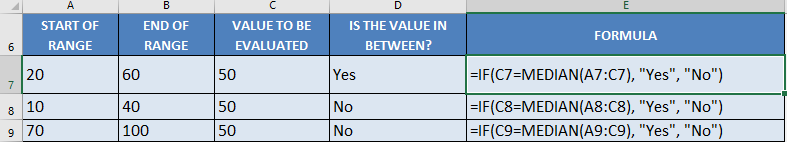## =IF(C7=MEDIAN(A7:C7), “Yes”, “No”)

In our first example above, the range is 20-60, upon checking the value 50, it is in between this range.
The median formula will return the value in the middle of these 3 values when arranged in increasing order: 20, 50, 60. The median value is 50. Since it matches the value we are evaluating, then the answer we get is a Yes, this value (50) is in between the range.
STEP 2: For dates, we have the same application of the median formula. Because Excel treats dates as numbers too:

## =IF(C10=MEDIAN(A10:C10), “Yes”, “No”)

In our first example above, the range is May 1 – July 1, upon checking the date June 1, it is in between this range.
The median formula will return the value in the middle of these 3 dates when arranged in increasing order: May 1, June 1, July 1. The median value is June 1. Since it matches the value we are evaluating, then the answer we get is a Yes, this value (June 1) is in between the range.
STEP 3: For text, we are checking if the value is alphabetically in the middle. We will be using the and formula: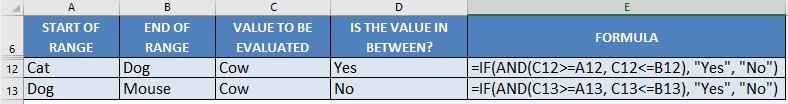## =IF(AND(C12>=A12, C12<=B12, "Yes", "No")

Interestingly enough, you can compare texts using the >= and <= operators.  Excel is able to compare them which goes alphabetically first or last.
In our first example above, the range is Cat – Dog, upon checking the text Cow, it is in between this range. As when arranged alphabetically, it would be: Cat, Cow, Dog.
The And formula checks if Cow >= Cat, and Cow <= Dog. You will see that both of these are true, as Cow is alphabetically later than Cat, while Cow is alphabetically ahead of Dog. Which is why we get a Yes result.

## FILTER Formula in Excel

What does it do?
Filters a table array based on the filtering condition given
Formula breakdown:
=FILTER(array, include, [if_empty])
What it means:
=FILTER(data to be filtered, the filtering condition, [value to display if nothing gets matched])

Did you know that you can now filter your table data with an Excel Formula? Yes you can! It is definitely possible now with Excel’s FILTER Formula. It is a new formula introduced in Office 365 released in 2018!
We have a tax table that we want to dynamically filter with a given rate.

I explain how you can do this below:

STEP 1: We need to enter the FILTER function in a blank cell:

## =FILTER(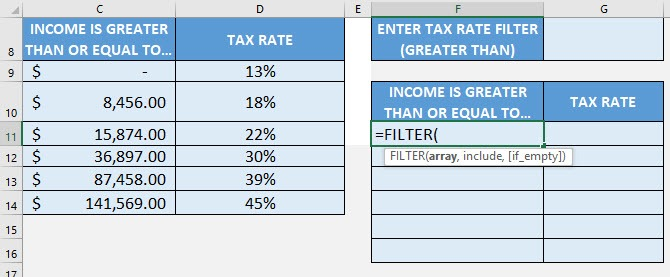STEP 2: The FILTER arguments:

## array

What is the data to be filtered?
Select the cells containing the tax data, do not include the headers:

## =FILTER(C9:D14,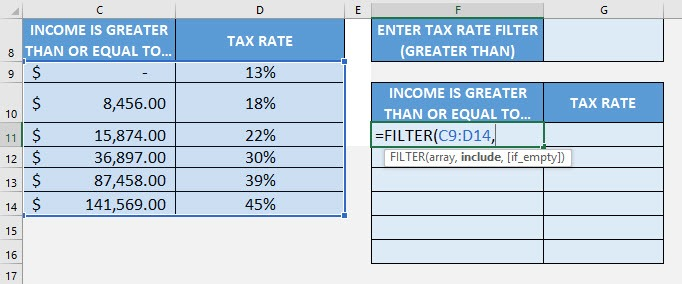## include

We want to filter the tax rate that is greater than the specified rate. Type in the condition as the tax rate column > the specific tax rate.

## =FILTER(C9:D14, D9:D14>G8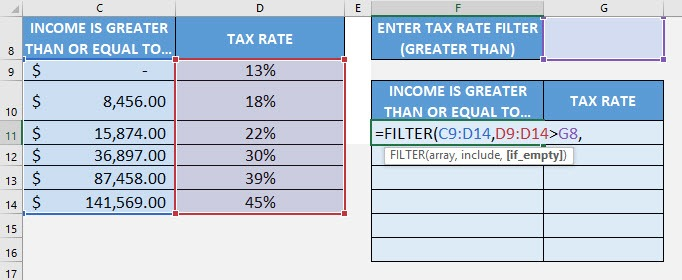## [if_empty]

What is the value to display in case nothing gets matched?
Just place an empty string to be displayed if nothing gets matched.

## =FILTER(C9:D14, D9:D14>G8, “”)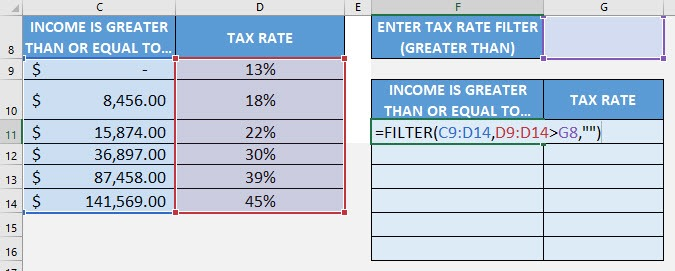Try it out now with different values and see it get filtered magically!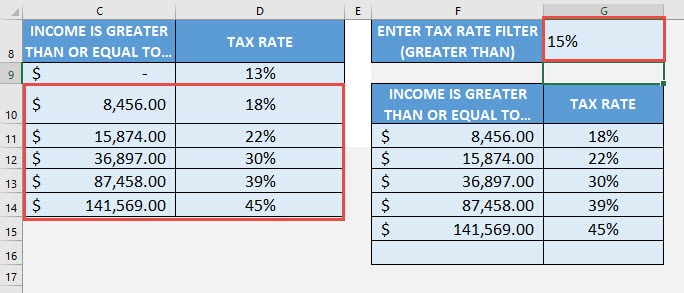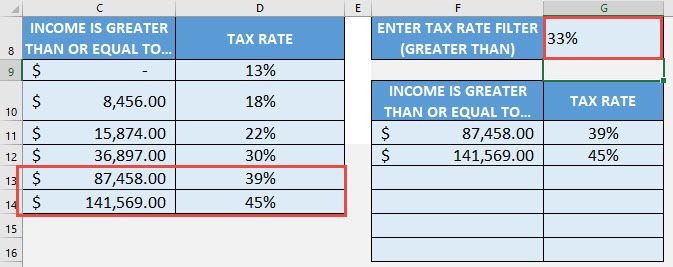## SWITCH Formula in Excel

What does it do?
Matches multiple values and returns the first value that has a match
Formula breakdown:
=SWITCH(expression, value1, result1, [value2 / default, result2], …)
What it means:
=SWITCH(value to check, value to match against, result to return, [succeeding values to match or the default value if nothing gets matched], …)

If you have multiple values to check, we can use Excel’s SWITCH Formula! It allows us to specify multiple values to check, then the SWITCH Formula will look for the first value that gets matched!
Let us try it out on a simple ratings table (e.g. 1 = Bad, 2 = Average, 3 = Great), then we will create a SWITCH Formula that will simulate the exact same logic of the table!
I explain how you can do this below:

STEP 1: We need to enter the SWITCH function in a blank cell:

## =SWITCH(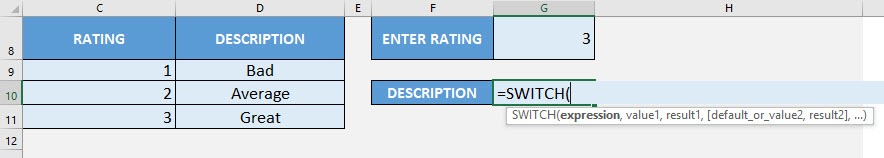STEP 2: The SWITCH arguments:

## expression

What is the value to check?
Select the cell containing the rating that you want to translate to the correct description

## =SWITCH(G8,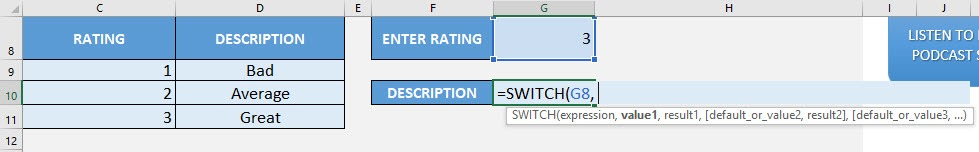## value1, result1

What is the first lookup value and value to return if it is matched?
Let us start from the first value of the rating table. If the value is 1, then the description is “Bad”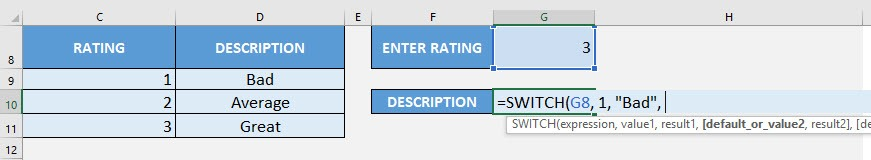## value2, result2

What is the second lookup value and value to return if it is matched?
Let us start from the second value of the rating table. If the value is 2, then the description is “Average”

## =SWITCH(G8, 1, “Bad”, 2, “Average”,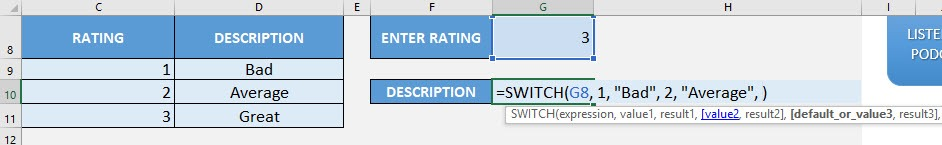## value3, result3

What is the third lookup value and value to return if it is matched?
Let us start from the third value of the rating table. If the value is 3, then the description is “Great”

## =SWITCH(G8, 1, “Bad”, 2, “Average”, 3, “Great”,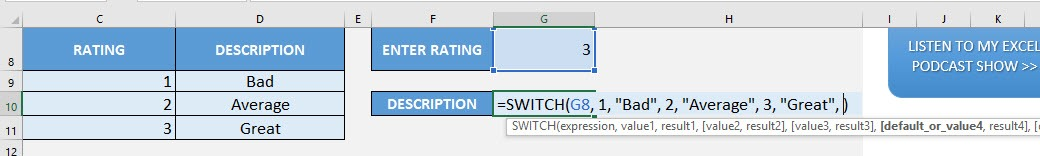## default

What is the default value to return if nothing gets matched?
We want to show the value “Unknown”, if an unknown rating is specified.

## =SWITCH(G8, 1, “Bad”, 2, “Average”, 3, “Great”, “Unknown”)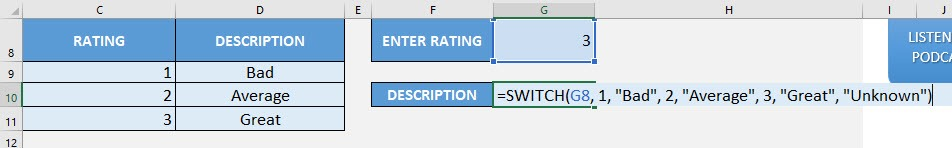You now have your correct rating description!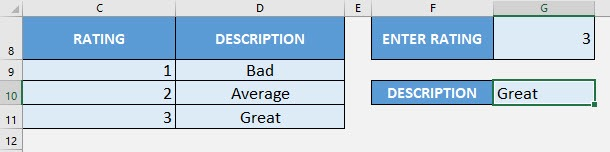Let us try an unknown rating (40) and see the resulting description: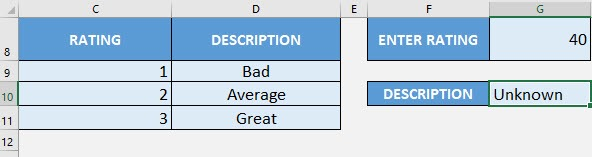Latest Tutorials

June 7, 2021

March 3, 2021